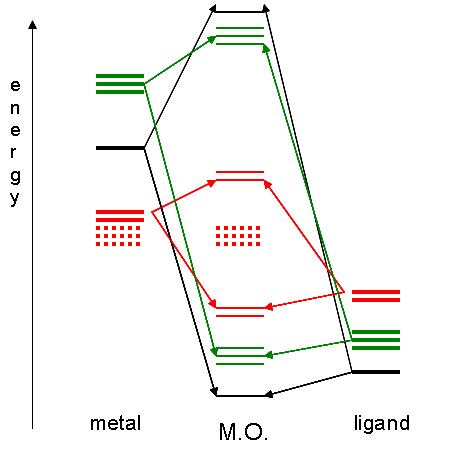# Metals, Tetrahedral and Octahedral

$$\newcommand{\vecs}{\overset { \rightharpoonup} {\mathbf{#1}} }$$ $$\newcommand{\vecd}{\overset{-\!-\!\rightharpoonup}{\vphantom{a}\smash {#1}}}$$$$\newcommand{\id}{\mathrm{id}}$$ $$\newcommand{\Span}{\mathrm{span}}$$ $$\newcommand{\kernel}{\mathrm{null}\,}$$ $$\newcommand{\range}{\mathrm{range}\,}$$ $$\newcommand{\RealPart}{\mathrm{Re}}$$ $$\newcommand{\ImaginaryPart}{\mathrm{Im}}$$ $$\newcommand{\Argument}{\mathrm{Arg}}$$ $$\newcommand{\norm}{\| #1 \|}$$ $$\newcommand{\inner}{\langle #1, #2 \rangle}$$ $$\newcommand{\Span}{\mathrm{span}}$$ $$\newcommand{\id}{\mathrm{id}}$$ $$\newcommand{\Span}{\mathrm{span}}$$ $$\newcommand{\kernel}{\mathrm{null}\,}$$ $$\newcommand{\range}{\mathrm{range}\,}$$ $$\newcommand{\RealPart}{\mathrm{Re}}$$ $$\newcommand{\ImaginaryPart}{\mathrm{Im}}$$ $$\newcommand{\Argument}{\mathrm{Arg}}$$ $$\newcommand{\norm}{\| #1 \|}$$ $$\newcommand{\inner}{\langle #1, #2 \rangle}$$ $$\newcommand{\Span}{\mathrm{span}}$$$$\newcommand{\AA}{\unicode[.8,0]{x212B}}$$Figure 1: The d (left) and dx²−y² (right) orbitals. Images used with permission from Wikipedia.

Let us continue to consider an octahedral complex. The remaining d orbitals, dxy, dxz and dyz see their energy increase to a lesser extent. We refer to the dxy, dxz and dyz orbitals collectively as the t2g d orbitals.

Hybrid orbital theory can be used to describe how metals bond to ligands. When metals bond to ligands, magnetic data shows that some electrons are paired when there is no obvious reason for them to be paired. Molecular orbitals begin to account for this phenomenon by allowing wave functions to interfere in a constructive, low energy (bonding) or destructive, or high energy (antibonding) manner. Thus, the electrons can fill the lowest energy molecular orbitals available to them. However, the electron pairing may be different if the electrons were allowed to fill the lowest energy atomic orbitals available to them.This diagram shows the field splitting of a metal with ligands in an octahedral configuration. The thick horizontal lines represent atomic orbitals of the metal (left) and ligands (right). The colors correspond to s (black), p (green) and d (red) orbitals. The middle column of horizontal lines represents molecular orbitals made of bonding (lower energy) and antibonding (higher energy) components.

If the ligands are oriented on the cartesian coordinate axes, the metal will still own 3 d orbitals, xy, xz, and xz, which do not intersect (and therefore do not interact) with the ligands. These are considered "nonbonding" orbitals, and are represented by dotted lines in the diagram.

## References

1. Jean, Yves; "molecular orbitals of transition metal complexes"; Oxford University Press, 2005